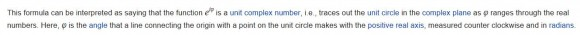## complex exponential - what Am I missing?Started by 6 years ago6 replieslatest reply 6 years ago141 views

The following is from Wikipedia - https://en.wikipedia.org/wiki/Euler%27s_formulabelow is what I get when I try for 0 and 30 deg

>> sqrt(cos(0)+sin(0))
ans =  1
>> sqrt(cos(30*2*pi/360)+sin(30*2*pi/360))
ans =  1.1688

Should I not be expecting ans = 1 for every value of theta?

[ - ]Hi.  To reinforce what UliBru wrote, your

sqrt(cos(30*2*pi/360)+sin(30*2*pi/360))

command should be changed to:

sqrt(cos(30*2*pi/360)^2 + sin(30*2*pi/360)^2)

[ - ]Apologies. That was really stupid of me

[ - ]Sharan123, don't worry.  The only people who never makes mistakes are people who never do anything.  :-)

[ - ]If your goal is to understand Euler's equation, you might find my first blog article helpful:

"The Exponential Nature of the Complex Unit Circle"

https://www.dsprelated.com/showarticle/754.php

Ced

[ - ]Cedron,

Thanks. I will take a look.

[ - ]